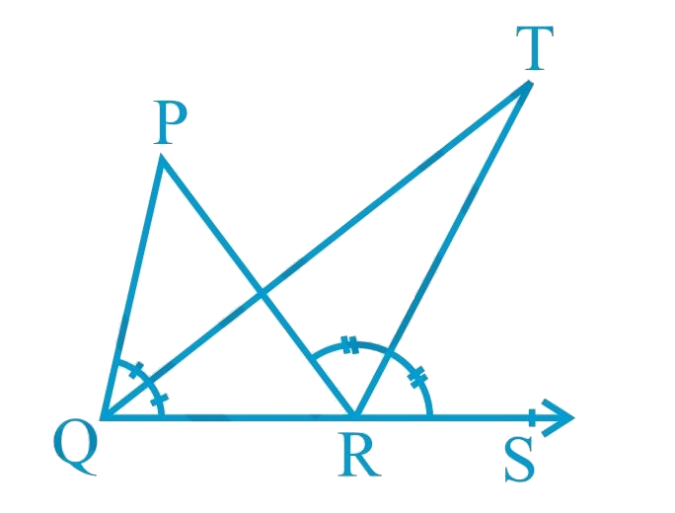# Ex.6.3 Q6 Lines and Angles Solution - NCERT Maths Class 9

## Question

In Fig. below, the side $$QR$$ of $$\Delta PQR$$ is produced to a point $$S$$. If the bisectors of $$\angle PQR$$ and $$\angle PRS$$ meet at point $$T$$, then prove that \begin{align} \angle QTR = \frac{1}{2}\angle QPR\end{align} .## Text Solution

What is known?

The side $$QR$$ of $$\Delta PQR$$ is produced to a point $$S$$ and the bisectors of $$\angle PQR$$ and $$\angle PRS$$ meet at point $$T$$.

To prove:

\begin{align}\angle QTR = \frac{1}{2}\angle QPR\end{align}

Reasoning:

As we know exterior angle of a triangle:

If a side of a triangle is produced, then the exterior angle so formed is equal to the sum of the two interior opposite angles.

Steps:

Given,

Bisectors of $$\angle PQR$$ and $$\angle PRS$$ meet at point $$T$$.

Hence, $$TR$$ is a bisector of $$\angle PRS$$ and $$TQ$$ is a bisector of $$\angle PQR$$

\begin{align} \angle PRS &= 2\angle TRS\quad\dots\left( {\rm{i}} \right)\\\angle PQR &= 2\angle TQR\quad\dots\left( {{\rm{ii}}} \right)\end{align}

Now, in $$\Delta TQR$$

\begin{align}\angle TRS&= \angle TQR + \angle QTR\\ &\left( {{\text{Exterior angle of triangle}}} \right)\\\\\angle QTR &= \angle TRS-\angle TQR\quad\dots\left( {{\text{iii}}} \right)\end{align}

Similarly, in $$\Delta PQR$$

\begin{align}\angle PRS &= \angle QPR + \angle PQR\qquad\\ &\left( {{\text{Exterior angle of triangle}}} \right)\\\\2\angle TRS &= \angle QPR + 2\angle TQR\\ &\left[ {{\text{From }}\left( {\rm{i}} \right){\rm{ and }}\left( {{\rm{ii}}} \right)} \right]\\\\\angle QPR &= 2\angle TRS-2\angle TQR\\\angle QPR &= 2\left( {\angle TRS-\angle TQR} \right)\\\angle QPR &= 2\angle QTR\qquad\qquad\,\,\,\,\,\qquad\\ &\left[ {{\text{From }}\left( {{\rm{iii}}} \right)} \right]\\\\\angle QTR &= \frac{1}{2}\angle QPR\end{align}

Hence proved.

Learn from the best math teachers and top your exams

• Live one on one classroom and doubt clearing
• Practice worksheets in and after class for conceptual clarity
• Personalized curriculum to keep up with school